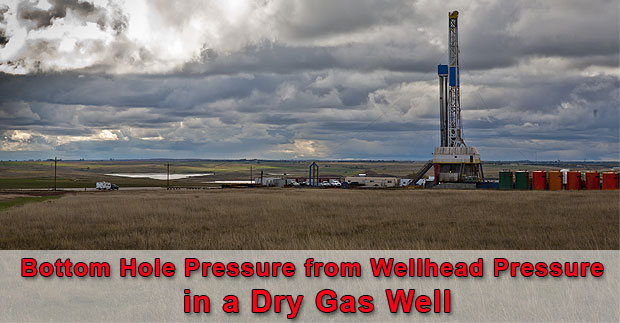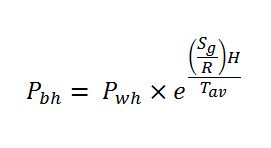## Determine Bottom Hole Pressure from Wellhead Pressure in a Dry Gas Well

Gas behaves differently from fluid therefore you cannot use a simple hydrostatic formula to determine reservoir pressure. Gas is compressible but fluid is incompressible.The formula to determine the bottom hole pressure of dry gas well is shown below;Where; Pbh = bottom hole pressure in psia (absolute pressure)

Pwh = wellhead pressure in psia (absolute pressure)

H = true vertical depth of the well

Sg = specific gravity of gas

R = 53.36 ft-lb/lb-R (gas constant for API standard condition air)

Tav = average temperature in Rankin (Rankin = Fahrenheit + 460) Continue reading